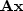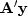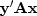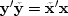There is a huge gap between the conception of an idea and putting it into practice. During development, things fail far more often than not. Often, when something fails, many tests are needed to track down the cause of failure. Maybe the cause cannot even be found. More insidiously, failure may be below the threshold of detection and poor performance suffered for years. I find the dot-product test to be an extremely valuable checkpoint.

Conceptually, the idea of matrix transposition is simply aij'=aji. In practice, however, we often encounter matrices far too large to fit in the memory of any computer. Sometimes it is also not obvious how to formulate the process at hand as a matrix multiplication. What we find in practice is that an application and its adjoint amounts to two subroutines. The first subroutine amounts to the matrix multiplication.The adjoint subroutine computes,whereis the transpose matrix. In a later chapter we will be solving huge sets of simultaneous equations. Then both subroutines are required. We are doomed from the start if the practitioner provides an inconsistent pair of subroutines. The dot product test is a simple test for verifying that the two subroutines are adjoint to each other.

The associative property of linear algebra says that we do not need parentheses in a vector-matrix-vector product likebecause we get the same result no matter where we put the parentheses. They serve only to determine the sequence of computation. Thus,(7) (8)
(In general, the matrix is not square.) For the dot-product test, load the vectorsandwith random numbers. Compute the vectorusing your program for,and computeusing your program for.Inserting these into equation (8) gives you two scalars that should be equal.(9)
The left and right sides of this equation will be computationally equal only if the program doingis indeed adjoint to the program doing(unless the random numbers do something miraculous).

I tested (9) on many operators and was surprised and delighted to find that it is often satisfied to an accuracy near the computing precision. More amazing is that on some computers, equation (9) was sometimes satisfied down to and including the least significant bit. I do not doubt that larger rounding errors could occur, but so far, every time I encountered a relative discrepancy of 10-5 or more, I was later able to uncover a conceptual or programming error. Naturally, when I do dot-product tests, I scale the implied matrix to a small dimension in order to speed things along, and to be sure that boundaries are not overwhelmed by the much larger interior.

Do not be alarmed if the operator you have defined has truncation errors. Such errors in the definition of the original operator should be identically matched by truncation errors in the adjoint.

If your code passes the dot-product test, then you really have coded the adjoint operator. In that case, you can take advantage of the standard methods of mathematics to obtain inverse operators.

We can speak of a continuous function f(t) or a discrete one ft. For continuous functions we use integration, and for discrete ones we use summation. In formal mathematics the dot-product test defines the adjoint operator, except that the summation in the dot product may need to be changed to an integral. The input or the output or both can be given either on a continuum or in a discrete domain. So the dot-product testcould have an integration on one side of the equal sign and a summation on the other. Linear-operator theory is rich with concepts, but I will not develop it here. I assume that you studied it before you came to read this book, and that it is my job to show you how to use it.